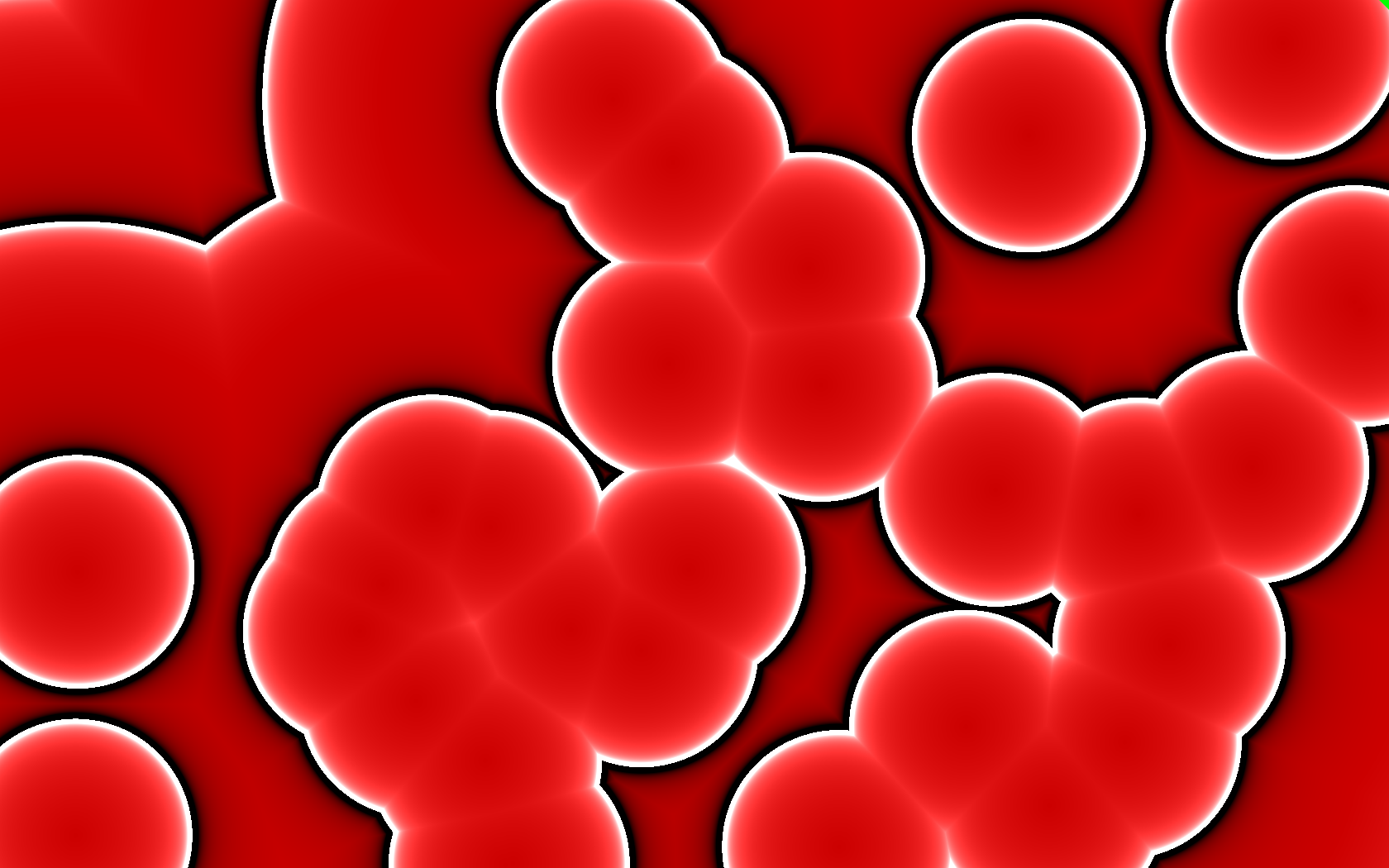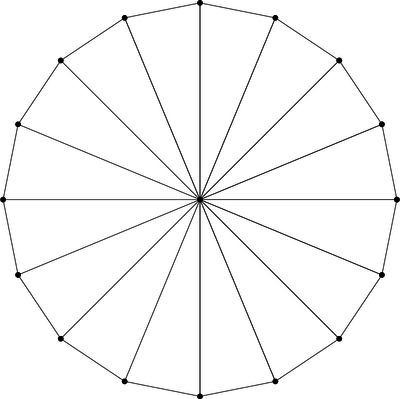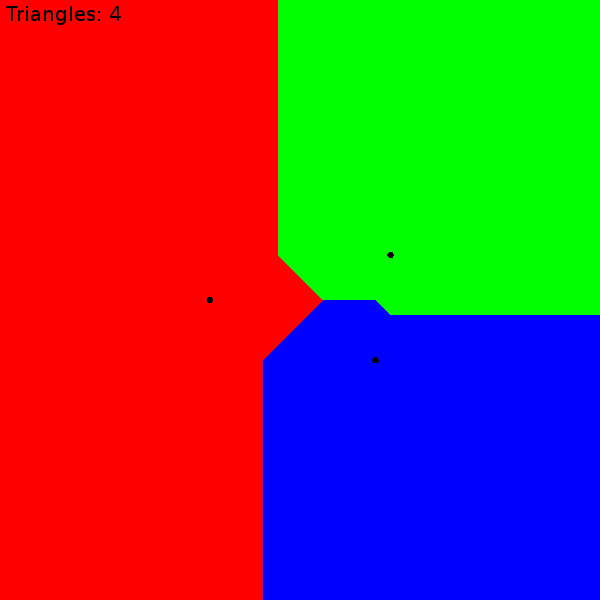## A GPU Approach to Voronoi Diagrams

I recently got an itch to play around with Voronoi diagrams. It’s a diagram that divides a space into regions composed of points closest to one of a set of seed points. There are a couple of algorithms for computing a Voronoi diagram: Bowyer-Watson and Fortune. These are complicated and difficult to implement.

However, if we’re interested only in rendering a Voronoi diagram as a bitmap, there’s a trivial brute for algorithm. For every pixel of output, determine the closest seed vertex and color that pixel appropriately. It’s slow, especially as the number of seed vertices goes up, but it works perfectly and it’s dead simple!

Does this strategy seem familiar? It sure sounds a lot like an OpenGL fragment shader! With a shader, I can push the workload off to the GPU, which is intended for this sort of work. Here’s basically what it looks like.

``````/* voronoi.frag */
uniform vec2 seeds;
uniform vec3 colors;

void main() {
float dist = distance(seeds, gl_FragCoord.xy);
vec3 color = colors;
for (int i = 1; i < 32; i++) {
float current = distance(seeds[i], gl_FragCoord.xy);
if (current < dist) {
color = colors[i];
dist = current;
}
}
gl_FragColor = vec4(color, 1.0);
}
``````

If you have a WebGL-enabled browser, you can see the results for yourself here. Now, as I’ll explain below, what you see here isn’t really this shader, but the result looks identical. There are two different WebGL implementations included, but only the smarter one is active. (There’s also a really slow HTML5 canvas fallback.)

You can click and drag points around the diagram with your mouse. You can add and remove points with left and right clicks. And if you press the “a” key, the seed points will go for a random walk, animating the whole diagram. Here’s a (HTML5) video showing it off.

Unfortunately, there are some serious problems with this approach. It has to do with passing seed information as uniforms.

1. The number of seed vertices is hardcoded. The shader language requires uniform arrays to have known lengths at compile-time. If I want to increase the number of seed vertices, I need to generate, compile, and link a new shader to replace it. My implementation actually does this. The number is replaced with a `%%MAX%%` template that I fill in using a regular expression before sending the program off to the GPU.

2. The number of available uniform bindings is very constrained, even on high-end GPUs: `GL_MAX_FRAGMENT_UNIFORM_VECTORS`. This value is allowed to be as small as 16! A typical value on high-end graphics cards is a mere 221. Each array element counts as a binding, so our shader may be limited to as few as 8 seed vertices. Even on nice GPUs, we’re absolutely limited to 110 seed vertices. An alternative approach might be passing seed and color information as a texture, but I didn’t try this.

3. There’s no way to bail out of the loop early, at least with OpenGL ES 2.0 (WebGL) shaders. We can’t `break` or do any sort of branching on the loop variable. Even if we only have 4 seed vertices, we still have to compare against the full count. The GPU has plenty of time available, so this wouldn’t be a big issue, except that we need to skip over the “unused” seeds somehow. They need to be given unreasonable position values. Infinity would be an unreasonable value (infinitely far away), but GLSL floats aren’t guaranteed to be able to represent infinity. We can’t even know what the maximum floating-point value might be. If we pick something too large, we get an overflow garbage value, such as 0 (!!!) in my experiments.

Because of these limitations, this is not a very good way of going about computing Voronoi diagrams on a GPU. Fortunately there’s a much much better approach!

### A Smarter Approach

With the above implemented, I was playing around with the fragment shader, going beyond solid colors. For example, I changed the shade/color based on distance from the seed vertex. A results of this was this “blood cell” image, a difference of a couple lines in the shader.That’s when it hit me! Render each seed as cone pointed towards the camera in an orthographic projection, coloring each cone according to the seed’s color. The Voronoi diagram would work itself out automatically in the depth buffer. That is, rather than do all this distance comparison in the shader, let OpenGL do its normal job of figuring out the scene geometry.

Here’s a video (GIF) I made that demonstrates what I mean.

Not only is this much faster, it’s also far simpler! Rather than being limited to a hundred or so seed vertices, this version could literally do millions of them, limited only by the available memory for attribute buffers.

#### The Resolution Catch

There’s a catch, though. There’s no way to perfectly represent a cone in OpenGL. (And if there was, we’d be back at the brute force approach as above anyway.) The cone must be built out of primitive triangles, sort of like pizza slices, using `GL_TRIANGLE_FAN` mode. Here’s a cone made of 16 triangles.Unlike the previous brute force approach, this is an approximation of the Voronoi diagram. The more triangles, the better the approximation, converging on the precision of the initial brute force approach. I found that for this project, about 64 triangles was indistinguishable from brute force.#### Instancing to the Rescue

At this point things are looking pretty good. On my desktop, I can maintain 60 frames-per-second for up to about 500 seed vertices moving around randomly (“a”). After this, it becomes draw-bound because each seed vertex requires a separate glDrawArrays() call to OpenGL. The workaround for this is an OpenGL extension called instancing. The WebGL extension for instancing is `ANGLE_instanced_arrays`.

The cone model was already sent to the GPU during initialization, so, without instancing, the draw loop only has to bind the uniforms and call draw for each seed. This code uses my Igloo WebGL library to simplify the API.

``````var cone = programs.cone.use()
.attrib('cone', buffers.cone, 3);
for (var i = 0; i < seeds.length; i++) {
cone.uniform('color', seeds[i].color)
.uniform('position', seeds[i].position)
.draw(gl.TRIANGLE_FAN, 66);  // 64 triangles == 66 verts
}
``````

It’s driving this pair of shaders.

``````/* cone.vert */
attribute vec3 cone;
uniform vec2 position;

void main() {
gl_Position = vec4(cone.xy + position, cone.z, 1.0);
}
``````
``````/* cone.frag */
uniform vec3 color;

void main() {
gl_FragColor = vec4(color, 1.0);
}
``````

Instancing works by adjusting how attributes are stepped. Normally the vertex shader runs once per element, but instead we can ask that some attributes step once per instance, or even once per multiple instances. Uniforms are then converted to vertex attribs and the “loop” runs implicitly on the GPU. The instanced glDrawArrays() call takes one additional argument: the number of instances to draw.

``````ext = gl.getExtension("ANGLE_instanced_arrays"); // only once

programs.cone.use()
.attrib('cone', buffers.cone, 3)
.attrib('position', buffers.positions, 2)
.attrib('color', buffers.colors, 3);
/* Tell OpenGL these iterate once (1) per instance. */
ext.vertexAttribDivisorANGLE(cone.vars['position'], 1);
ext.vertexAttribDivisorANGLE(cone.vars['color'], 1);
ext.drawArraysInstancedANGLE(gl.TRIANGLE_FAN, 0, 66, seeds.length);
``````

The ugly ANGLE names are because this is an extension, not part of WebGL itself. As such, my program will fall back to use multiple draw calls when the extension is not available. It’s only there for a speed boost.

Here are the new shaders. Notice the uniforms are gone.

``````/* cone-instanced.vert */
attribute vec3 cone;
attribute vec2 position;
attribute vec3 color;

varying vec3 vcolor;

void main() {
vcolor = color;
gl_Position = vec4(cone.xy + position, cone.z, 1.0);
}
``````
``````/* cone-instanced.frag */
varying vec3 vcolor;

void main() {
gl_FragColor = vec4(vcolor, 1.0);
}
``````

On the same machine, the instancing version can do a few thousand seed vertices (an order of magnitude more) at 60 frames-per-second, after which it becomes bandwidth saturated. This is because, for the animation, every vertex position is updated on the GPU on each frame. At this point it’s overcrowded anyway, so there’s no need to support more.